# Coach

The average age of 24 players and a coach of one team is 24 years. The average age of players without a coach is 23 years. How old is the coach?

t =  48

### Step-by-step explanation:

(24•23+t)/25 = 24

t = 48

Our simple equation calculator calculates it.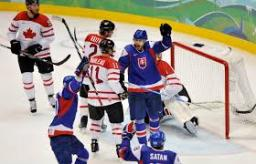Did you find an error or inaccuracy? Feel free to write us. Thank you!Tips to related online calculators
Looking for help with calculating arithmetic mean?
Looking for a statistical calculator?
Do you have a linear equation or system of equations and looking for its solution? Or do you have a quadratic equation?

#### You need to know the following knowledge to solve this word math problem:

We encourage you to watch this tutorial video on this math problem:

## Related math problems and questions:

• Team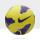The football team has 11 players. Their average age is 20 years. During the match due to injury, one player withdraws and the average age of the team is 18. How old was injured player?
• Average ageThe average age of all people at the celebration was equal to the number of people present. After the departure of one person who was 29 years old, average age was again equal to the number present. How many people were originally to celebrate?
• Average ageThe company of five people has an average age of 46 years. The average age of the first four is 43 years. How many years has the fifth member of this company?
• Large familyThe average age of all family members (children, mother, father, grandmother, grandfather) is 29 years. The average age of parents is 40 years, grandparents 66 years and all children are 5 years. How many children are there in this family?
• GrandfatherThe grandfather is three years older than a grandmother. The average age of the grandmother, the grandfather, and their seven grandchildren is 28 years. The grandchildren have a total of 105 years. Calculate how old grandfather is.
• Players - baseball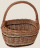There are 20 players on each of two baseball teams. If 2/5 of the players on team 1 miss practice and 1/4 of the players on team two miss practice, how many more players from team 1 missed practice then team 2?
• Young mathematician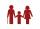One young mathematician was bored again. He found that the average age of people in the room where the seminar equals to its count. Then his 29-year-old brother entered this room. Even then, the average age of all present was the same as the count of peop
• TroopsThere are two leaders in the tourist group, with an average age of 30 years and several children with an average age of 10 years. The total age of the group is 12 years. How many children are in the group?
• Average mark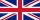Calculate average mark in English, if a student got a couple of 3's, 20% less 2 than 3, and 50% more 1's than the 2's.
• Average speedThe truck drove 1/2 of the way on the highway at 80km/h. The other half of the way 20km/h. Calculate the average speed
• Age problemsA) Alex is 3 times as old as he was 2 years ago. How old is he now? b) Casey was twice as old as his sister 3 years ago. Now he is 5 years older than his sister. How old is Casey? c) Jessica is 4 years younger than Jennifer now. In 10 years, Jessica will
• Mortality tables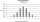Mortality tables enable actuaries to obtain the probability that a person at any age will live a specified number of years. Insurance companies and others use such probabilities to determine life-insurance premiums, retirement pensions, and annuity paymen
• Maya is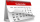Maya is 14 years old. Her brother Jorge is 3 years more than half her age. Which of the following is the correct expression for Jorge's age?
• PeroxideHow many ml should we pour 30% of peroxide (H2O2) into 100ml H2O to give a 20% solution?
• Age of familyThe age of father and son is in the ratio 10: 3. The age of the father and daughter is in a ratio of 5: 2. How old are a father and a son if the daughter is 20?
• Salary in enterprise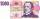The average salary in the company is 27 000 CZK, 30% of workers have the lowest average income of 19 thousand CZK. There were an increase in the salary in this group by 2%. How much % increased the average salary across the company?
• Family and age ratiosThe age of father and son are in the ratio 10:3; the age of father and daughter are in the ratio 5:2. How old are father and son if the daughter is 20 years old? In what ratio is age sister and brother?